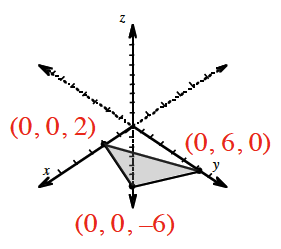### Home > INT3 > Chapter 12 > Lesson 12.2.2 > Problem12-101

12-101.

Consider the equation $3x + y - z = 6$.

1. Sketch a graph.

Notice that there are three variables. Find the three intercepts.2. Is $\left(1, 2, –1\right)$ on the graph? Justify your answer.

Does the point make the equation true?

Yes, it is a solution to the equation.